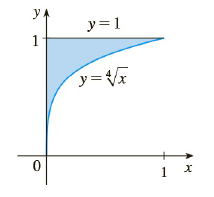Chapter 4.4, Problem 46E

Chapter
Section
Textbook Problem

# The boundaries of the shaded region in the figure are the y-axis, the line y = 1, and the curve y = x 4 . Find the area of this region by writing x as a function of y and integrating with respect to y (as in Exercise 45).To determine

To find:

The area of the region.

Solution:

15

Explanation

1) Concept:

The fundamental theorem of calculus part 2: If  f  is continuous on a,b, then abfxdx=Fb-Fa,  where  F  is any antiderivative of f , that is, a function  F  such that F'=f .

2) Formula:

fx+gxdx=fxdx+gxdx

xndx=xn+1n+1+C

3) Given:

y=x4 ;from y=0 to</

### Still sussing out bartleby?

Check out a sample textbook solution.

See a sample solution

#### The Solution to Your Study Problems

Bartleby provides explanations to thousands of textbook problems written by our experts, many with advanced degrees!

Get Started

#### Find more solutions based on key concepts